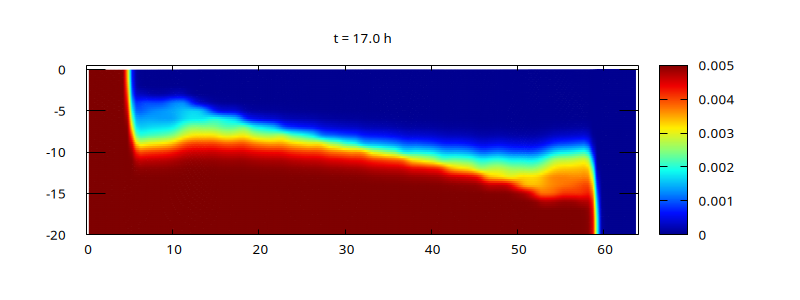# Lock exchange

This is the test case proposed by Ilicak et al, 2012 to estimate numerical mixing. The parameters (spatial resolution, horizontal and vertical viscosities) match those of Ilicak et al, section 3.Density perturbation field at t=17 hours

The density pertubation field at t=17 hours can be compared to the equivalent results in the last row of Figure 2 of Ilicak et al, 2012, for three different solvers: ROMS, MITgcm and MOM.

The evolution of the normalised reference potential energy below can be compared with Figure 4.a (solid lines) of Ilicak et al. As expected the variation is small and comparable to that of ROMS, which also uses a \sigma-coordinate in the vertical.

set xlabel 'Time (hours)'
set ylabel '(RPE - RPE(0))/RPE(0) x 10^5'
hour = 3600.
set xrange [0:17]
plot 'log' u ($1/hour):(-$3/$4*1e5) w l t ''Evolution of the normalised reference potential energy (script) set ylabel 'dRPE/dt (Watts/m^2)' set logscale y L0 = 64e3 plot 'log' u ($1/hour):(abs($3)/$1/L0) w l t ''Evolution of the average rate of change of the reference potential energy (script)

set ylabel 'APE (Joules)'
unset logscale y
plot 'log' every 1 u ($1/hour):($5-$4) w l t ''Evolution of the Available Potential Energy (APE). (script) set ylabel 'KE (Joules)' plot 'log' every 1 u ($1/hour):6 w l t ''Evolution of the Kinetic Energy. (script)

 [ilicak2012] Mehmet Ilicak, Alistair J. Adcroft, Stephen M. Griffies, and Robert W. Hallberg. Spurious dianeutral mixing and the role of momentum closure. Ocean Modelling, 45-46:37–58, 2012. [ DOI | http ]

## Code

The setup is almost identical to the overflow case which you should consult for a more detailed description.

#include "grid/multigrid1D.h"
#include "layered/hydro.h"
#include "layered/implicit.h"
#define rho0 1000.
#define drho(T) ((T))
#include "layered/dr.h"
#if ISOPYCNAL
# define HALF 1
#endif
#include "layered/remap.h"
#include "layered/rpe.h"

double nu_H = 0.01; // 200; // 0.01;

int main()
{
size (64e3);
N = 128;
nl = 20;
cell_lim = mono_limit;
G = 9.81;
DT = 60;
nu = 1e-4;

const scalar l[] = HUGE; // free slip
lambda_b = l;

run();
}

event init (i = 0)
{
foreach() {
zb[] = -20;
#if ISOPYCNAL
foreach_layer() {
T[] = point.l < nl/2 ? 0.005 : 0;
if (x > L0/2.)
h[] = point.l >= nl/2 ? 20./(nl/2) : 1e-4;
else
h[] = point.l <  nl/2 ? 20./(nl/2) : 1e-4;
}
#else
foreach_layer() {
h[] = 20./nl;
T[] = x < L0/2. ? 0.005 : 0;
}
#endif
}
}

event viscous_term (i++)
horizontal_diffusion ({u.x}, nu_H, dt);

event logfile (i += 10)
{
static double rpe0 = 0., rpen = 0., tn = 0.;
double rpe = rho0*RPE();
double PE, KE;
energy (&PE, &KE);
if (i == 0)
rpe0 = rpe;
fprintf (stderr, "%g %g %.12g %.12g %.12g %.12g %.12g %d\n", t, dt,
rpe - rpe0, rpe0, rho0*PE, rho0*KE,
t > tn ? (rpe - rpen)/(t - tn)/L0 : 0.,
#if NH
mgp.i
#else
mgH.i
#endif
);
rpen = rpe, tn = t;
}

void plot (FILE * fp)
{
fprintf (fp,
"set title 't = %.1f h'\n"
"sp [%g:%g][-20:0.5]'-' u (\$1/1e3):2:4\n",
t/3600., X0/1e3, (X0 + L0)/1e3);
foreach (serial) {
double z = zb[];
fprintf (fp, "%g %g %g %g\n", x, z, u.x[], T[]);
foreach_layer() {
z += h[];
fprintf (fp, "%g %g %g %g\n", x, z, u.x[], T[]);
}
fprintf (fp, "\n");
}
fprintf (fp, "e\n\n");
//  fprintf (fp, "pause .01\n");
fflush (fp);
}

event gnuplot (i += 10)
{
static FILE * fp = popen ("gnuplot 2> /dev/null", "w");
if (i == 0)
fprintf (fp,
"set term x11\n"
"set pm3d map corners2color c2\n"
"# jet colormap\n"
"set palette defined ( 0 0 0 0.5647, 0.125 0 0.05882 1, 0.25"
" 0 0.5647 1, 0.375 0.05882 1 0.9333, 0.5 0.5647 1 0.4392, 0.625"
" 1 0.9333 0, 0.75 1 0.4392 0, 0.875 0.9333 0 0, 1 0.498 0 0 )\n"
"unset key\n"
//	     "set cbrange [0:30]\n"
"set xlabel 'x (km)'\n"
"set ylabel 'depth (m)'\n"
);
plot (fp);
}

event pictures (t += 3600; t <= 17*3600)
{
FILE * fp = popen ("gnuplot 2> /dev/null", "w");
fprintf (fp,
"set term pngcairo font \",10\" size 800,300\n"
"set pm3d map interpolate 4,4\n"
"# jet colormap\n"
"set palette defined ( 0 0 0 0.5647, 0.125 0 0.05882 1, 0.25"
" 0 0.5647 1, 0.375 0.05882 1 0.9333, 0.5 0.5647 1 0.4392, 0.625"
" 1 0.9333 0, 0.75 1 0.4392 0, 0.875 0.9333 0 0, 1 0.498 0 0 )\n"
"unset key\n"
//	   "set cbrange [5:30]\n"
"set output 'T-%g.png'\n", t/3600);
plot (fp);
}